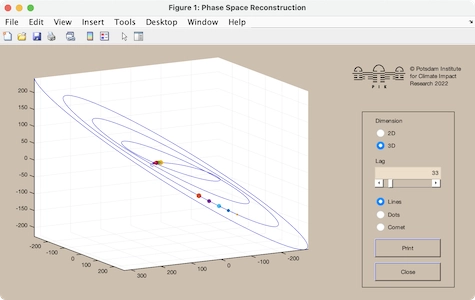# CRP Toolbox

## phasespace

Embedding of time series in a phase space.

### Syntax

``````phasespace(x)
phasespace(x,y)
phasespace(x,y,z)
``````

### Description

Shows the 3D phase space trajectory of the system which is presented by the observation x. The phase vectors are a reconstruction by using the time delay method (Takens, 1981). A GUI provides to change the embedding dimension to 2D.

phasespace(x,y) or phasespace(x,y,z) uses the one-column vectors x, y (and z) as the components of the phase space trajectory. The representation is 2D (3D) only and cannot be switched to the other representation.

phasespace without any arguments calls a demo (the same as the example below).

### Example

``````phasespace(cos(0:.1:32).*[321:-1:1])
``````